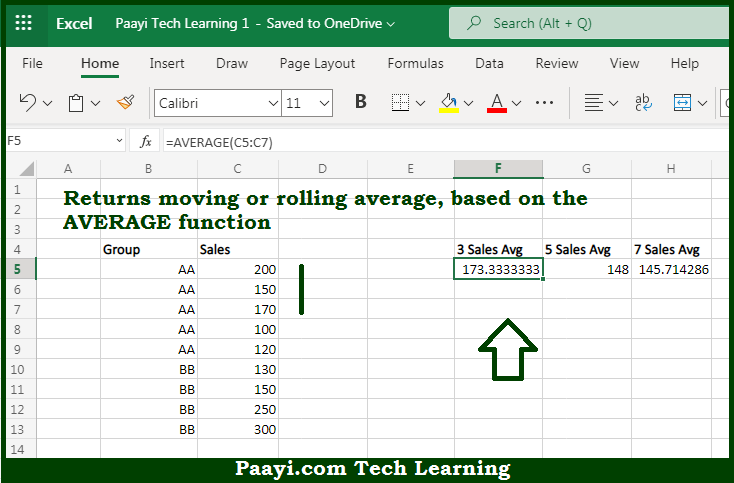# Learn How to Moving AVERAGE Formula in Microsoft Excel

Written by | 0 Comments | 456 Views

In this article, you will learn how to Moving AVERAGE Formula in Microsoft Excel using a single/combination(s) of functions. You will also know how to Moving the AVERAGE Formula and see the generic formula.

Moving AVERAGE Formula in Microsoft Excel

The main purpose of this formula is to calculate a moving or rolling average. Here we will learn how to get the moving of given data range based on the multiple criteria in the workbook in Microsoft Excel. That implies, with the help of a formula based on the AVERAGE function you can able to calculate a moving or rolling average. So, with the help of this formula, you can able to get the moving of given data range based on the multiple criteria in the workbook in Microsoft Excel.

General Formula to Moving AVERAGE Formula

=AVERAGE(Range)

The Explanation for the Moving AVERAGE FormulaSo we know that with the help of the given formula above you can able to calculate a moving or rolling average. Here we will learn how to get the moving of given data range based on the multiple criteria in the workbook in Microsoft Excel. As we know that the moving average also called a rolling average is an average based on subsets of data at given intervals. Calculating an average at specific intervals simplifies the data by reducing the impact of random fluctuations. That further makes simpler to analyze a trend, especially in a chart. The larger the interval used to calculate a moving average, the more smoothing that occurs since more data points are included in each calculated average. So, with the help of this formula, you can able to get the moving of given data range based on the multiple criteria in the workbook in Microsoft Excel.